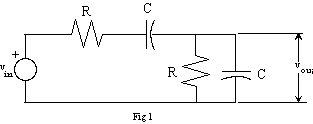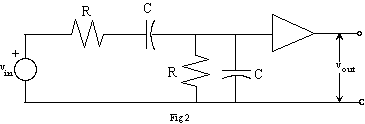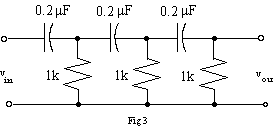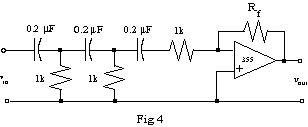EE 212 Lab
Lab 12: Wien Bridge and Phase Shift Oscillators

The importance of oscillators in electronics is illustrated in this excerpt from The Art of Electronics by Horowitz and Hill:

Within nearly every electronic instrument it is essential to have an oscillator or waveform generator of some sort. Apart from the obvious cases of signal generators, function generators, and pulse generators themselves, a source of regular oscillations is necessary in any cyclical measuring instrument, in any instrument that initiates measurements or processes, and in any instrument whose function involves periodic states or periodic waveforms. For example, oscillators or waveform generators are used in digital multimeters, oscilloscopes, radiofrequency receivers, computers, every computer peripheral (tape, disc, printer, alphanumeric terminal), nearly every digital instrument (counters, timers, calculators, and anything with a "multiplexed display''), and a host of other devices too numerous to mention. A device without an oscillator either doesn't do anything or expects to be driven by something else (which probably contains an oscillator). It is not an exaggeration to say that an oscillator of some sort is as essential an ingredient in electronics as a regulated supply of dc power.

Wien bridge and phase shift oscillators are good ways to produce low-distortion sinusoidal signals at low to moderate frequencies. These oscillators are circuits in which the output is equal to the input at a given frequency. For such a circuit, when the output is connected back to the input, a self-sustaining oscillation takes place at the given frequency.

1. Build the following circuit using the component values determined in the prelab. With a sinusoidal input, observe the phase shift between input and output. Find the frequency at which the phase shift is zero and compare to the design frequency of 10kHz. Determine the ratio of the output to the input amplitude and compare to the value calculated in the prelab.2. Connect the above circuit to a non-inverting amplifier based upon a 355/356 op-amp. This is depicted in figure 2 where its noted that the amplifier symbol (triangle) represents the non-inverting amplifier circuit. Adjust the gain of the amplifier and the frequency of the function generator until the amplitude and the phase of the output equal those of the input. (Include a precision (multi-turn) pot in the amplifier circuit to make the gain adjustable.)3. When the output is the same as the input, disconnect the signal generator and connect the output of the circuit to the input. With a little adjustment of the gain, the circuit should oscillate, producing a nice sine wave. Measure the frequency, and compare to the design frequency and the frequency measured in Part 1.

4. The RC network in Figure 3 can produce a phase shift of 180° at a desired frequency.  In the prelab,  you calculated this frequency for the component values given.  Build the circuit.  With a sinusoidal input, adjust the frequency of the function generator until the phase shift is 180°. Compare the frequency that yields 180° of phase to the frequency computed in the prelab. What is the gain of the circuit? How does this compare to your prelab result?5. To make an oscillator, we need 0° or 360° of phase shift. Supply the extra phase shift with an inverting op-amp amplifier. The op-amp circuit can also supply the gain needed to make the input and output equal. Design and build the amplifier with adjustable gain. (The final 1 kW should become the input resistor, as it effectively goes to ground in both cases - compare Figure 3 and Figure 4.) Adjust the gain and frequency until vout = vin.

6. Complete the oscillator by  again connecting the input to the output. Adjust the gain for an undistorted sine wave. Measure the frequency. How does this compare to the design frequency?